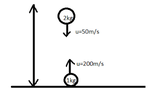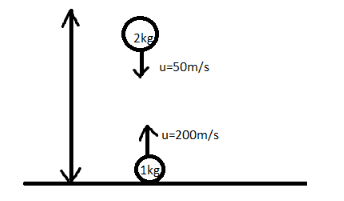# Motion of center of mass under gravity

• sachin
In summary, The balls stick together because it removes the possibility of one ball impacting the ground while the other continues moving upward. If such were to occur, that would be an unbalanced external force that would alter the velocity of the center of mass and could conceivably cause it to have a new, higher peak.f

#### sachin

Thread moved from the technical forums to the schoolwork forums
While solving this question I could not figure out the concept of two blocks sticking together.

the question is,
Two particles A and B of masses 1 kg and 2 kg respectively are projected in the directions shown in figure with speed uA =200m/s and uB =50m/s. Initially they were 90m apart. They collide in mid-air and stick with each other. Find the maximum height attained by the centre of mass of the particles.

while solving I am able to get the initial position and acceleration of the center of mass,

but I dnt know how to use the "the bodies collide and stick wih each other" given in the question,

I just understand that finally their velocities are equal but dnt kbow how to proceed further.

#### Attachments

•44cc05c3-6b35-40bf-8bfb-b077e7fecb612288744221610311919.png
2.3 KB · Views: 29
while solving I am able to get the initial position and acceleration of the center of mass
The "acceleration" of the center of mass ?

the solution what i have from online materials is as follows,they have never given the use of the bodies sticking together,

uA =200m/s
uB =50m/s
Initial distance between them is 90 m.
Now, Height of centre of mass=m1H1+m2H2m1+m2=m1H1+m2H2m1+m2
⇒200×0+90×22+1=1803=60m⇒200×0+90×22+1=1803=60m

Also, Velocity of centre of mass=m1V1+m2V2m1+m2=m1V1+m2V2m1+m2
⇒200×1−50×22+1=1003m/s⇒200×1−50×22+1=1003m/s

Now using the equation of motion,
v2=u2+2asv2=u2+2as
⇒02=(1003)2−2×10×h⇒02=(1003)2−2×10×h

Since, the final velocity has to be 0 to attain maximum height.
⇒h=100×1009×2×10=55.55m⇒h=100×1009×2×10=55.55m

Thus, the total height obtained by the centre of mass= (60+55.55) mThe maximum height attained by the centre of mass =115.55 m

Before the inelastic collision of A and B, the position of the "center of mass" is irrelevant. Try it by finding out where they collide, first.

The "acceleration" of the center of mass ?
acceleration of center of mass will be g, as using formulae a of c m = (m1a1 + m2a2)/(m1 + m2), a1 = a2= g (both fall freely under gravity), plugging in the formulae we will get a of c m = g.

Before the inelastic collision of A and B, the position of the "center of mass" is irrelevant. Try it by finding out where they collide, first.
This is irrelevant to the question.

the solution what i have from online materials is as follows,they have never given the use of the bodies sticking together
That would be because the sticking together is a red herring. The center of mass motion is unaffected by the internal forces of a system.

Now, Height of centre of mass=m1H1+m2H2m1+m2=m1H1+m2H2m1+m2
⇒200×0+90×22+1=1803=60m⇒200×0+90×22+1=1803=60m

Also, Velocity of centre of mass=m1V1+m2V2m1+m2=m1V1+m2V2m1+m2
⇒200×1−50×22+1=1003m/s⇒200×1−50×22+1=1003m/s

Now using the equation of motion,
v2=u2+2asv2=u2+2as
⇒02=(1003)2−2×10×h⇒02=(1003)2−2×10×h

Since, the final velocity has to be 0 to attain maximum height.
⇒h=100×1009×2×10=55.55m⇒h=100×1009×2×10=55.55m
This may be due to your formatting, but the speed of the center of mass of two objects moving along the same line cannot be larger than the speed of both objects. Hence, 1003 m/s cannot be the center of mass speed for two objects with speeds 200 m/s and 50 m/s, respectively.

Also, again, the point is that the sticking together is irrelevant to the solution of the problem. The collision, whether fully elastic, fully inelastic, or partially elastic, does not change the motion of the center of mass because it only affects the internal forces of the system.

••berkeman and PeroK
Also, again, the point is that the sticking together is irrelevant to the solution of the problem. The collision, whether fully elastic, fully inelastic, or partially elastic, does not change the motion of the center of mass because it only affects the internal forces of the system.
The one relevance of the balls sticking together is to remove, even before any calculation, the possibility that one ball impacts the ground while the other continues moving upward.

If such were to occur, that would be an unbalanced external force that would alter the velocity of the center of mass and could conceivably cause it to have a new, higher peak.

With an inelastic collision, this possibility is removed. We know that there is no external force until the combined mass impacts on the ground.